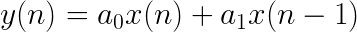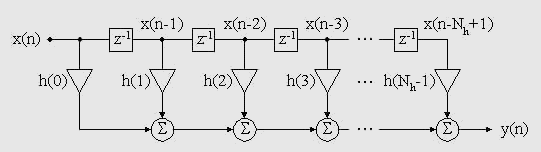In this article we will discuss direct-form convolution, which is perhaps the most straightforward approach to convolution; it also happens to be the most computationally inefficient methods for longer impulse responses. However, before any of that, it is important to discuss some basics about digital filtering.

# FILTERS

Without delving into endless DSP rabbit holes, a digital (audio) filter is a DSP block that accepts a discrete-time input signal, performs some sort of difference equation, and outputs a processed signal. Two types of digital filters exist: Infinite Impulse Response (IIR – feeds output signal back into input) and Finite Impulse Response (FIR – exclusively feeds input signal forward) filters; however, only FIR filters are relevant in this discussion.Mechanically, a simple first-order FIR filter just multiplies a set of coefficients with the input samples and various amounts of delay. The nature of the filter is described entirely by the coefficients; for example, a first order low-pass and high-pass filter can have exactly the same difference equation, but it’s the coefficients that determine the operation of the filter. Further: the set of the filter’s coefficient values are also the values in the filter’s impulse response.

# CONVOLUTION

The kicker here is when we bring this back to the discussion about convolution.FIR filters are applications of the above operation in which the coefficients of the filter are the amplitude samples of the impulse response. So theoretically, we could design an FIR filter whose coefficients were the samples of our cathedral’s impulse response and move any input signal through it, performing convolution. It would look like this:As you can see, especially for long impulse responses (longer than a second of audio sampled at 44.1 kHz), this approach is highly impractical. However, performing convolution this way can buy time while the next method “charges up” and then we can hand it off to a more efficient method that convolves the signals by entering the frequency domain. We will be looking at this more efficient approach next, including converting a signal into the frequency domain, performing circular convolution, and transforming the signal back into the time domain.